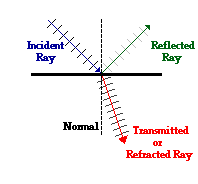Refraction and the Ray Model of Light - Lesson 3 - Total Internal Reflection

# Boundary Behavior RevisitedEarlier in this unit, the boundary behavior of light waves was discussed. It was mentioned that a light wave doesn't just stop when it reaches the end of the medium. Rather, the light wave undergoes certain behaviors when it encounters the end of the medium - such behaviors include reflection, transmission/refraction, and diffraction. In Unit 13 of The Physics Classroom Tutorial, the primary focus was the reflective behavior of light waves at the boundary. In this unit, our primary interest has been the refractive behavior of light waves at the boundary. In Lesson 3, we will investigate the connection between light reflection and light refraction.

A light wave, like any wave, is an energy-transport phenomenon. A light wave transports energy from one location to another. When a light wave strikes a boundary between two distinct media, a portion of the energy will be transmitted into the new medium and a portion of the energy will be reflected off the boundary and stay within the original medium. The actual percentage of energy that is transmitted and reflected is dependent upon a number of variables; these will be discussed as we proceed through Lesson 3. For now, our concern is to review and internalize the basic concepts and terminology associated with boundary behavior. Reflection of a light wave involves the bouncing of a light wave off the boundary, while refraction of a light wave involves the bending of the path of a light wave upon crossing a boundary and entering a new medium. Both reflection and refraction involve a change in direction of a wave, but only refraction involves a change in medium.

The diagram at the right shows several wavefronts approaching a boundary between two media. These wavefronts are referred to as the incident waves and the ray that points in the directionthat they are traveling is referred to as the incident ray. The incident ray is drawn in blue on the diagram at the right. Notice on the diagram that the incident ray leads into two other rays at the point of incidence with the boundary. The reflected waves are the waves that bounce off the boundary and head back upwards and the reflected ray is the ray that points in the direction that the reflected waves are traveling. The reflected ray is drawn in green on the diagram at the right. The refracted waves are the waves that are transmitted across the boundary and continue moving downwards, only at a different angle than before. The refracted ray is the ray that points in the direction that the refracted waves are traveling. The refracted ray is drawn in red on the diagram at the right. At the point of incidence (the point where the incident ray strikes the boundary), a normal line is drawn. The normal line is always drawn perpendicular to the surface at the point of incidence. The normal line creates a variety of angles with the light rays; these angles are important and are given special names. The angle between the incident ray and the normal is the angle of incidence. The angle between the reflected ray and the normal is the angle of reflection. And the angle between the refracted ray and the normal is the angle of refraction.

The fundamental law that governs the reflection of light is called the law of reflection. Whether the light is reflecting off a rough surface or a smooth surface, a curved surface or a planar surface, the light ray follows the law of reflection. The law of reflection states that

When a light ray reflects off a surface, the angle of incidence is equal to the angle of reflection.

The fundamental law that governs the refraction of light is Snell's Law. Snell's Law states that

When a light ray is transmitted into a new medium, the relationship between the angle of incidence (Θi) and the angle of refraction (Θr) is given by the following equation

ni • sine(Θi) = nr • sine(Θr)

where the ni and nr values represent the indices of refraction of the incident and the refractive medium respectively.

As we proceed through this Lesson, we will see that there is a connection between the reflection and the refraction of light. Each of these two behaviors usually occurs together. But as we will see, there are two conditions, which when both met, will cause the light waves to undergo reflection without any accompanying refraction.

### Flickr Physics Photo

Laser light shown passing into a hemi-cylindrical dish filled with water. The light enters the water (at the curved side of the dish) along the normal line; no bending occurs upon entry. The light continues through the water along a straight line until it reaches the boundary with air (at the flat side of the dish). The angle of incidence in the water is just short of 48°. Since the angle of incidence is just less than the air-water critical angle of about 48.6°, there is still a little refraction in addition to the reflection. The refracted ray can be seen at about 80°.### We Would Like to Suggest ...Why just read about it and when you could be interacting with it? Interact - that's exactly what you do when you use one of The Physics Classroom's Interactives. We would like to suggest that you combine the reading of this page with the use of our Refraction Interactive. You can find it in the Physics Interactives section of our website. The Refraction Interactive provides the learner an interactive enivronment for exploring the refraction and reflection of light at a boundary between two materials.

Next Section: